Learn All Concepts of Chapter 3 Class 12 Matrices - FREE. Check - Matrices Class 12 - Full video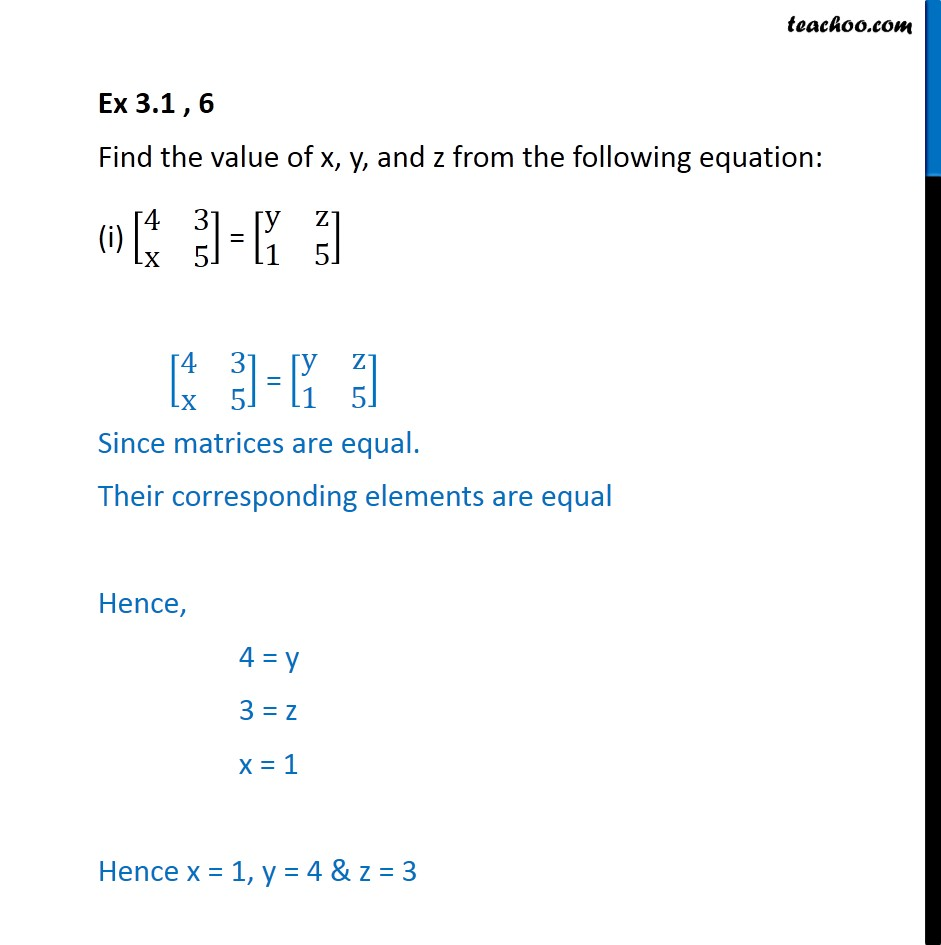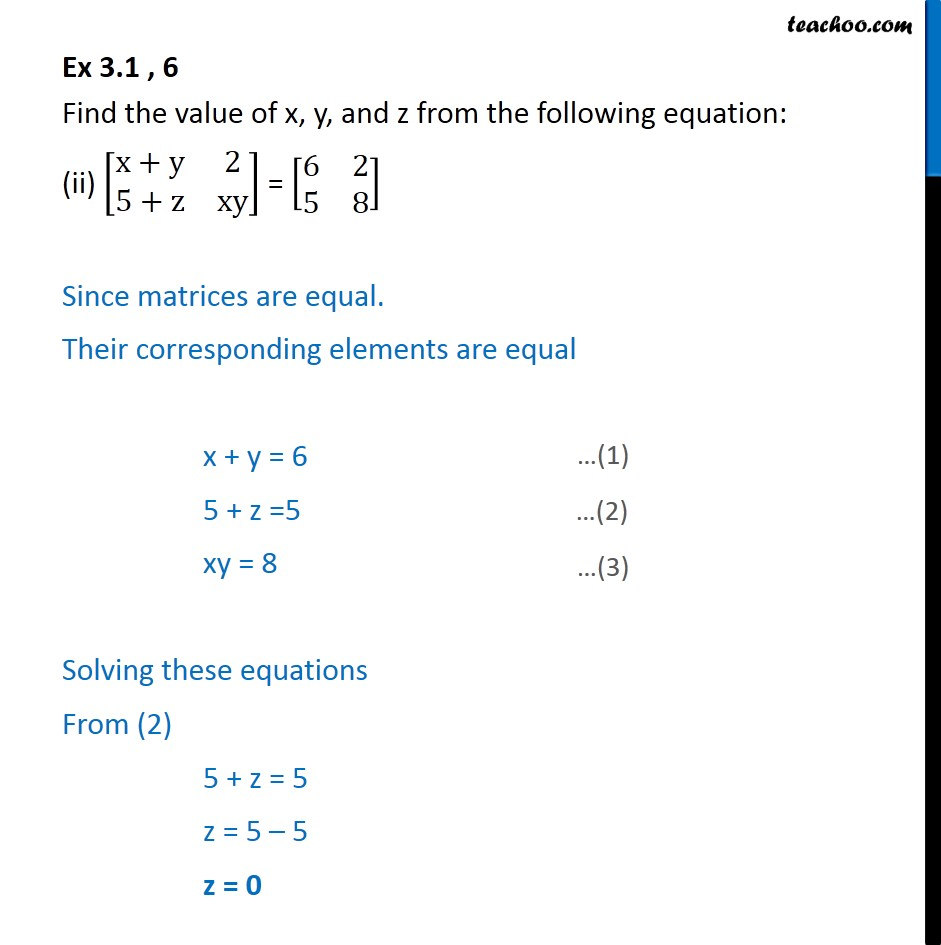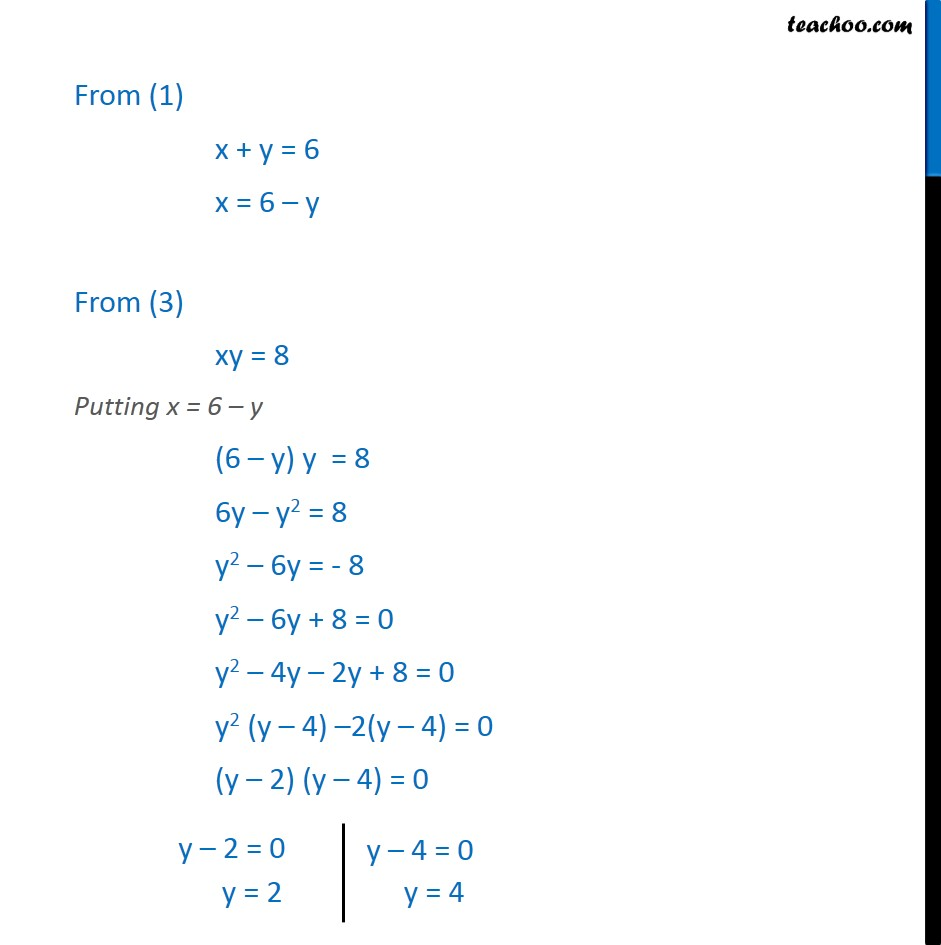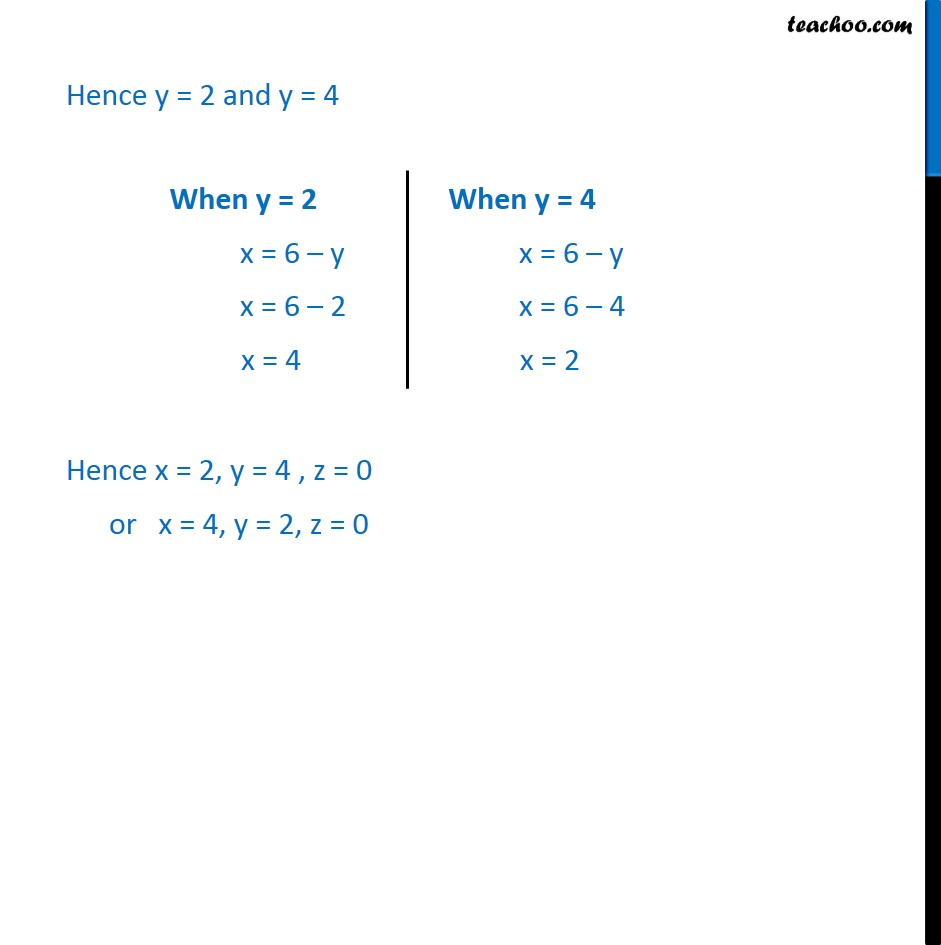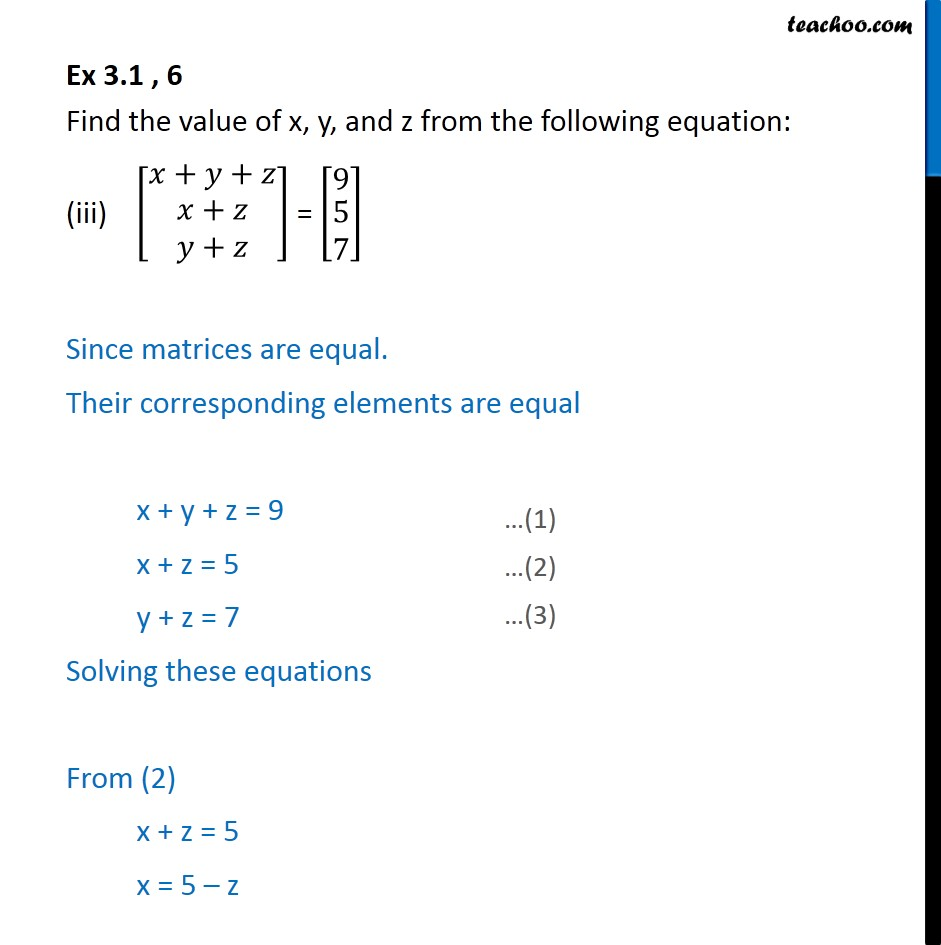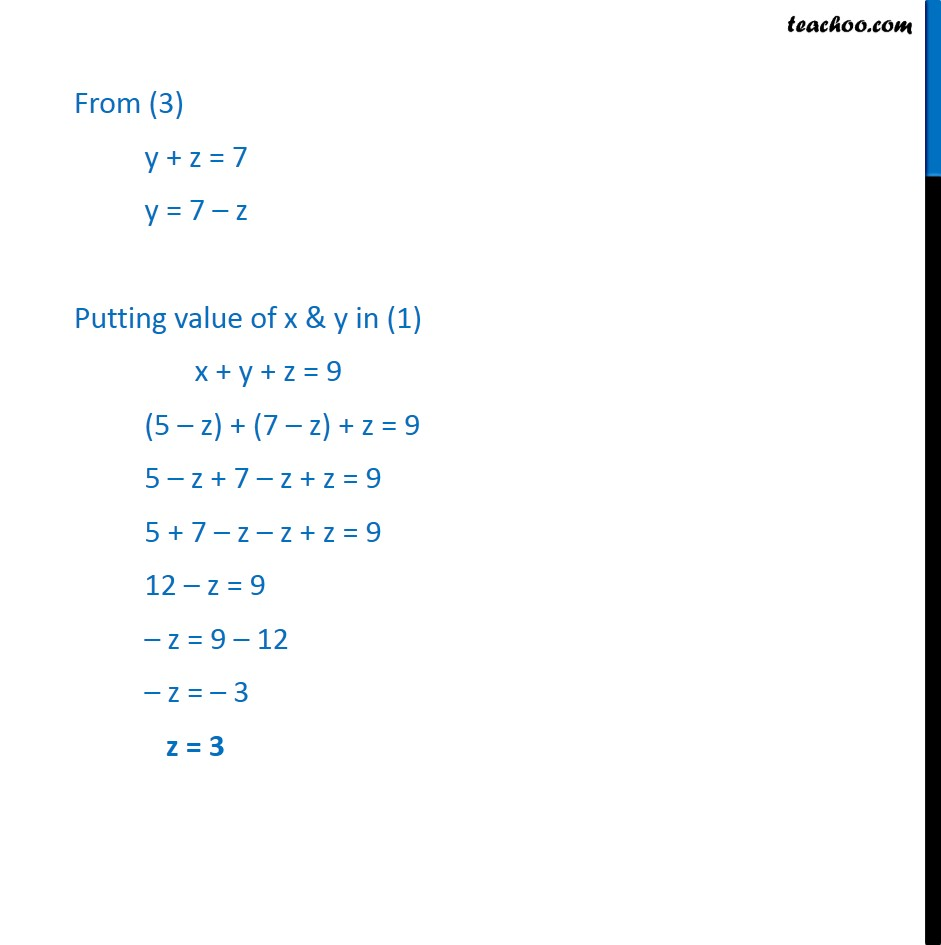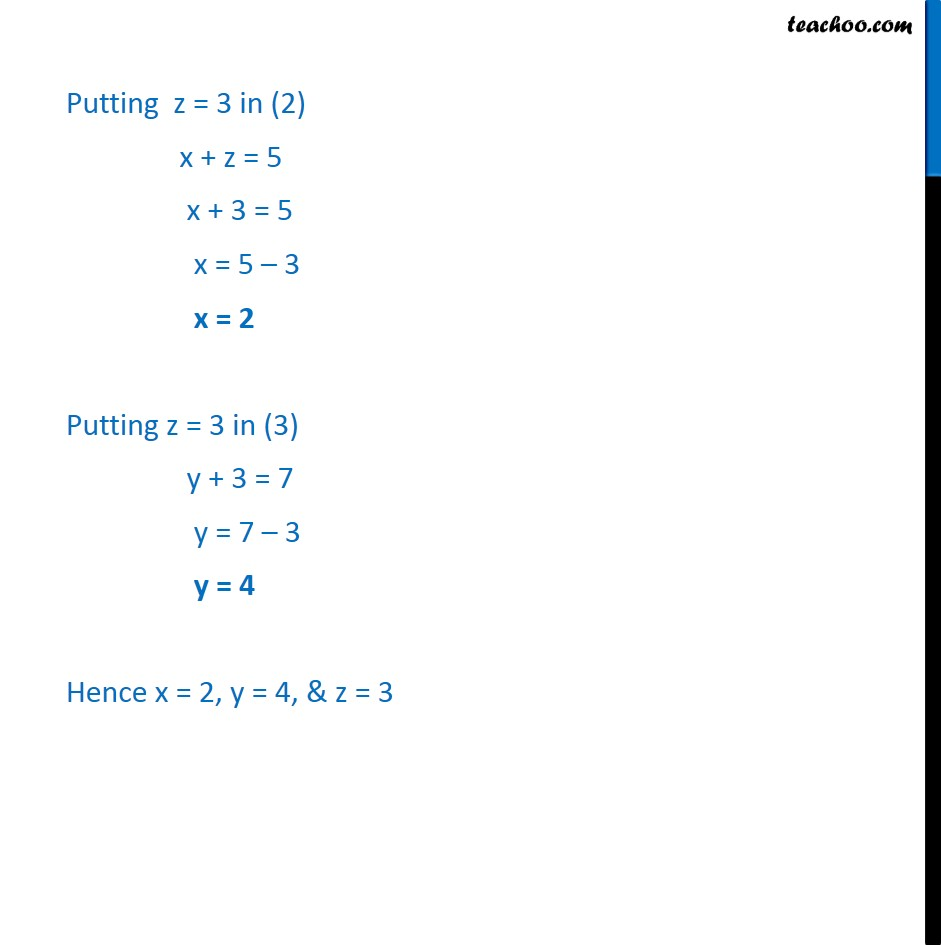1. Chapter 3 Class 12 Matrices
2. Serial order wise
3. Ex 3.1

Transcript

Ex 3.1 , 6 Find the value of x, y, and z from the following equation: (i) [ 8(4&3@x&5)] = [ 8(y&z@1&5)] [ 8(4&3@x&5)] = [ 8(y&z@1&5)] Since matrices are equal. Their corresponding elements are equal Hence, 4 = y 3 = z x = 1 Hence x = 1, y = 4 & z = 3 Ex 3.1 , 6 Find the value of x, y, and z from the following equation: (ii) [ 8(x+y&2@5+z&xy)] = [ 8(6&2@5&8)] Since matrices are equal. Their corresponding elements are equal x + y = 6 5 + z =5 xy = 8 Solving these equations From (2) 5 + z = 5 z = 5 5 z = 0 From (1) x + y = 6 x = 6 y From (3) xy = 8 Putting x = 6 y (6 y) y = 8 6y y2 = 8 y2 6y = - 8 y2 6y + 8 = 0 y2 4y 2y + 8 = 0 y2 (y 4) 2(y 4) = 0 (y 2) (y 4) = 0 Hence y = 2 and y = 4 Hence x = 2, y = 4 , z = 0 or x = 4, y = 2, z = 0 Ex 3.1 , 6 Find the value of x, y, and z from the following equation: (iii) [ 8( + + @ ( + @ + ))] = [ 8(9@ (5@7))] Since matrices are equal. Their corresponding elements are equal x + y + z = 9 x + z = 5 y + z = 7 Solving these equations From (2) x + z = 5 x = 5 z From (3) y + z = 7 y = 7 z Putting value of x & y in (1) x + y + z = 9 (5 z) + (7 z) + z = 9 5 z + 7 z + z = 9 5 + 7 z z + z = 9 12 z = 9 z = 9 12 z = 3 z = 3 Putting z = 3 in (2) x + z = 5 x + 3 = 5 x = 5 3 x = 2 Putting z = 3 in (3) y + 3 = 7 y = 7 3 y = 4 Hence x = 2, y = 4, & z = 3

Ex 3.1

Chapter 3 Class 12 Matrices
Serial order wise